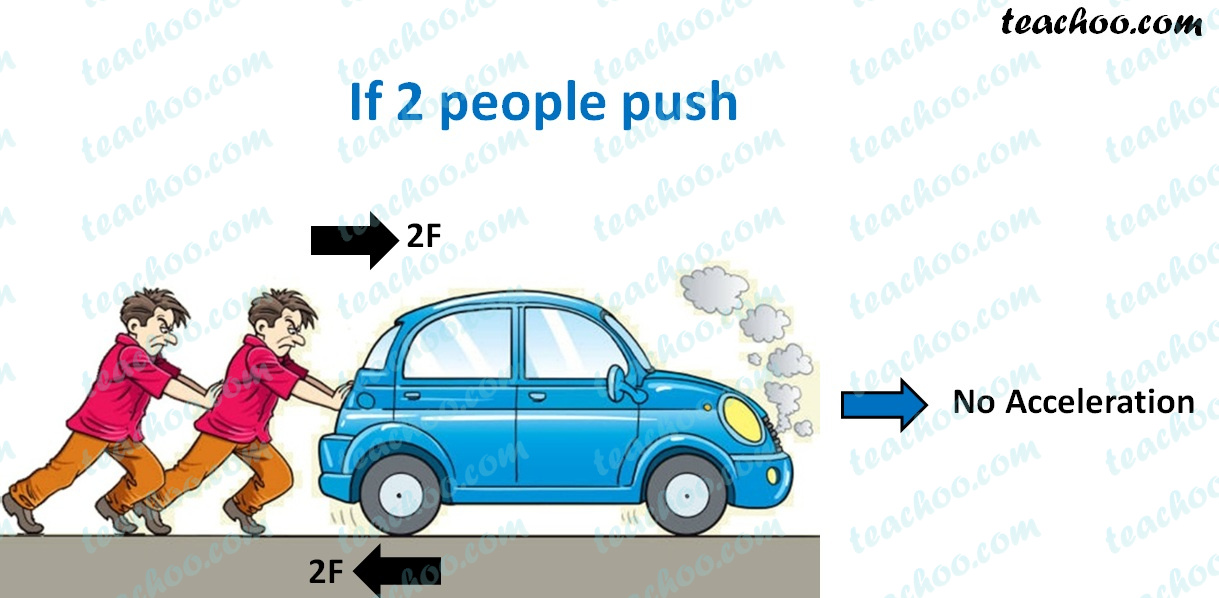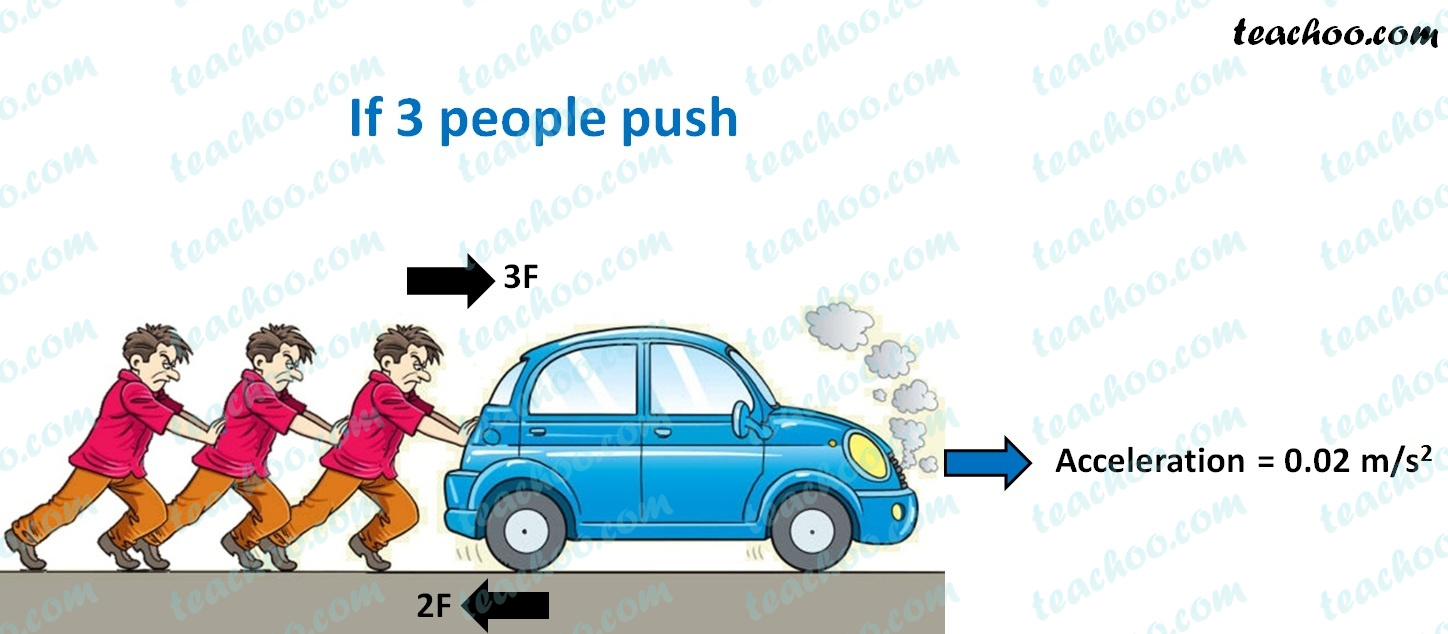Class 9
Chapter 9 Class 9 - Force and Laws Of Motion

## Two persons manage to push a motorcar of mass 1200 kg at a uniform velocity along a level road. The same motorcar can be pushed by three persons to produce an acceleration of 0.2 m s−2. With what force does each person push the motorcar? (Assume that all persons push the motorcar with the same muscular effort.)

If 2 people pushThen car moves with uniform velocity

So, Acceleration = 0

That means

Total Force on Body is balanced

Therefore,

Force by 2 People = Force of Friction

2F = Force of Friction

Force of Friction = 2F

If 3 people push

Image

Then car moves with Acceleration = 0.02 m/s2

Net Force = Force of 3 people – Force of Friction

= 3F – 2F

= FSo, let’s find F

Given

Mass of motor car = m = 1200 kg

Acceleration = a = 0.2 m/s2

Force = F

Now

Force = Mass × Acceleration

F = 1200 × 0.2

F = 1200 × 2/10

F = 120 × 2

F = 240N

Thus, each person pushes the motorcar by 240  force

Learn in your speed, with individual attention - Teachoo Maths 1-on-1 Class